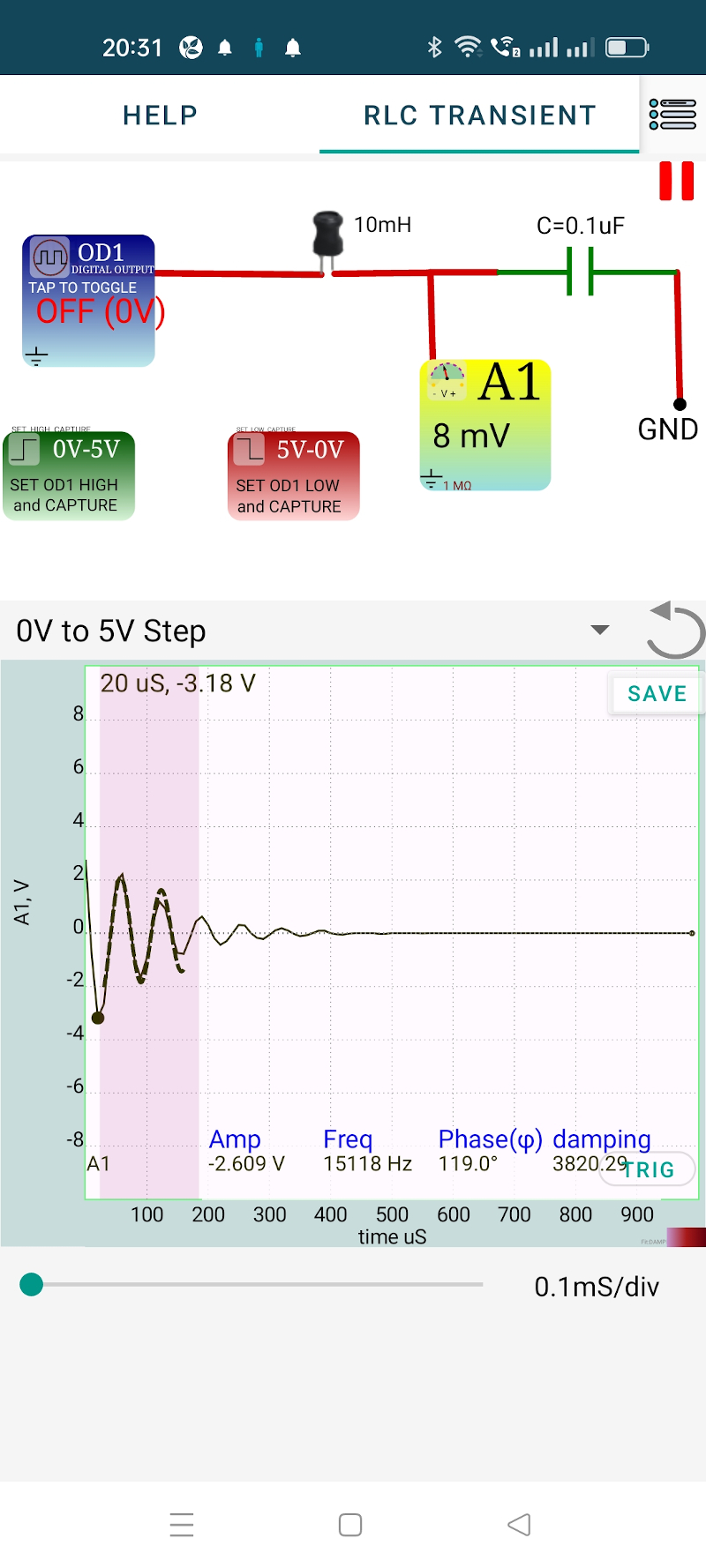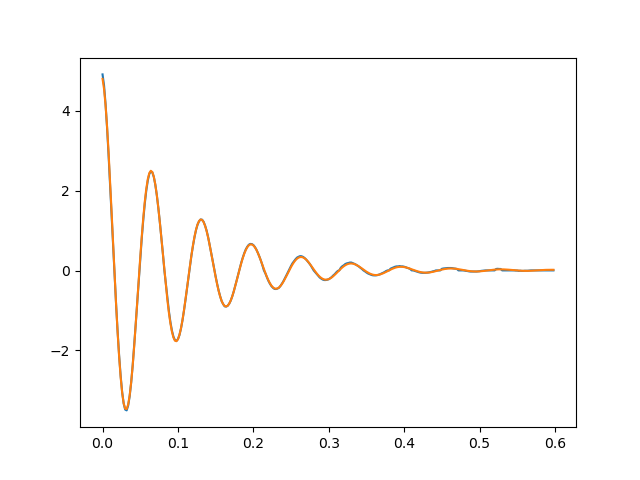# ExpEYES

## Transient Response of RLC circuits

The objecive is to study the evolution of voltage across the capacitor in response to a voltage step applied to the series circuit. For a theoretical discussion refer to the Online Course on ExpEYES. Depending on the LCR values, the response can be underdamped, critically damped or overdamped. We have selected:

• R = $100\Omega$
• L = 10 mH
• C = 10 nF

The connection diagram is shown in the schematic below. The trace can be fitted with a damped sinusoid by selecting a region. The data can be exported or saved. The exported data in CSV format is HERE. It can be analysed using this Python program to extract the frequency and damping factor.### By writing Python code

The experiment is repeated, with the same component values, by connecting ExpEYES to a laptop and using this Python program.

The data saved by this program is analysed by the Python Code and the result is shown below. Frequency = 15084 Hz and Damping factor = 0.107.

The equation $f = \frac{1}{2\pi \sqrt(LC)}$ gives 15915 Hz. For better agreement, we need to use measued component values in the calculation